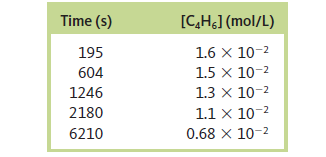# Problem: The dimerization of butadiene2C4H6 (g) → C8H12 (g)was studied at 500. K, and the following data were obtained:Assuming thatRate = - Δ [C4H6] / Δtdetermine the form of the rate law, the integrated rate law, and the value of the rate constant for this reaction.

99% (83 ratings)
###### Problem Details

2C4H6 (g) → C8H12 (g)

was studied at 500. K, and the following data were obtained:

Assuming thatRate = - Δ [C4H6] / Δt

determine the form of the rate law, the integrated rate law, and the value of the rate constant for this reaction.